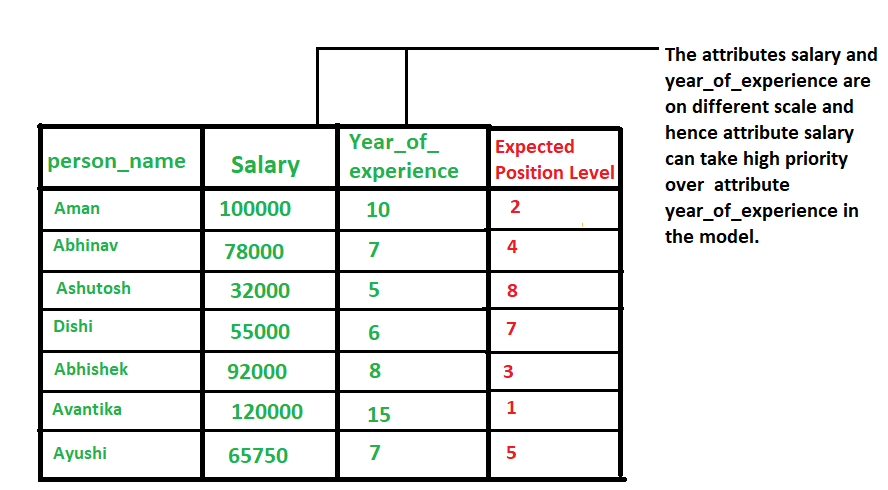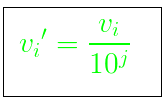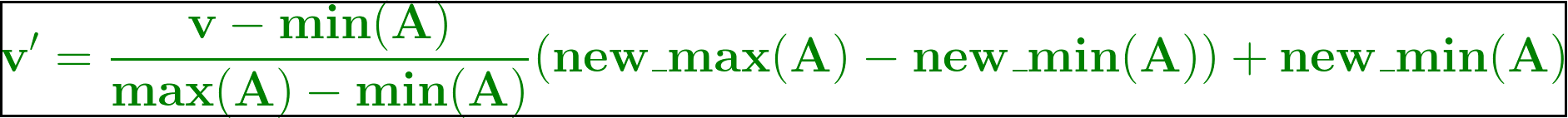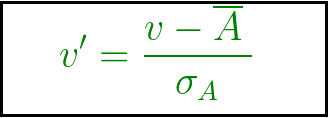Related Articles
Data Normalization in Data Mining
• Difficulty Level : Basic
• Last Updated : 25 Jun, 2019

Normalization is used to scale the data of an attribute so that it falls in a smaller range, such as -1.0 to 1.0 or 0.0 to 1.0. It is generally useful for classification algorithms.

### Need of Normalization –

Normalization is generally required when we are dealing with attributes on a different scale, otherwise, it may lead to a dilution in effectiveness of an important equally important attribute(on lower scale) because of other attribute having values on larger scale.
In simple words, when multiple attributes are there but attributes have values on different scales, this may lead to poor data models while performing data mining operations. So they are normalized to bring all the attributes on the same scale.Methods of Data Normalization –

• Decimal Scaling
• Min-Max Normalization
• z-Score Normalization(zero-mean Normalization)

### Decimal Scaling Method For Normalization –

It normalizes by moving the decimal point of values of the data. To normalize the data by this technique, we divide each value of the data by the maximum absolute value of data. The data value, vi, of data is normalized to vi by using the formula below –where j is the smallest integer such that max(|vi‘|)<1.

Example –

Let the input data is: -10, 201, 301, -401, 501, 601, 701

To normalize the above data,
Step 1: Maximum absolute value in given data(m): 701
Step 2: Divide the given data by 1000 (i.e j=3)

Result: The normalized data is: -0.01, 0.201, 0.301, -0.401, 0.501, 0.601, 0.701

### Min-Max Normalization –

In this technique of data normalization, linear transformation is performed on the original data. Minimum and maximum value from data is fetched and each value is replaced according to the following formula.Where A is the attribute data,
Min(A), Max(A) are the minimum and maximum absolute value of A respectively.
v’ is the new value of each entry in data.
v is the old value of each entry in data.
new_max(A), new_min(A) is the max and min value of the range(i.e boundary value of range required) respectively.

### Z-score normalization –

In this technique, values are normalized based on mean and standard deviation of the data A. The formula used is:v’, v is the new and old of each entry in data respectively. σA, A is the standard deviation and mean of A respectively.

Attention reader! Don’t stop learning now. Get hold of all the important CS Theory concepts for SDE interviews with the CS Theory Course at a student-friendly price and become industry ready.

My Personal Notes arrow_drop_up